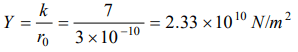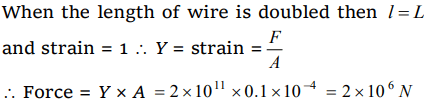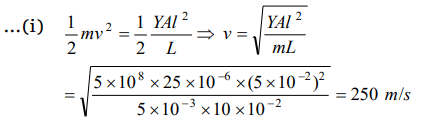## Mechanical Properties of Solids Questions and Answers Part-11

1. A uniform plank of Young’s modulus Y is moved over a smooth horizontal surface by a constant horizontal force F. The area of cross section of the plank is A. The compressive strain on the plank in the direction of the force is
a) $F\diagup AY$
b) $2F\diagup AY$
c) $\frac{1}{2}\left(F\diagup AY\right)$
d) $3F\diagup AY$

Explanation:2.The mean distance between the atoms of iron is $3\times 10^{-10}m$   and interatomic force constant for iron is 7 N/m The Young’s modulus of elasticity for iron is
a) $2.33\times 10^{5}N\diagup m^{2}$
b) $23.3\times 10^{10}N\diagup m^{2}$
c) $233\times 10^{10}N\diagup m^{2}$
d) $2.33\times 10^{10}N\diagup m^{2}$

Explanation:3. Two wires A and B are of same materials. Their lengths are in the ratio 1 : 2 and diameters are in the ratio 2 : 1 when stretched by force FA and FB respectively they get equal increase in their lengths. Then the ratio $F_{A}\diagup F_{B}$  should be
a) 1 : 2
b) 1 : 1
c) 2 : 1
d) 8 : 1

Explanation:4. The breaking stress of a wire depends upon
a) Length of the wire
c) Material of the wire
d) Shape of the cross section

Explanation: Material of the wire

5. The area of cross section of a steel wire $\left(Y=2.0\times 10^{11}N\diagup m^{2}\right)$    is 0.1 cm2. The force required to double its length will be
a) $2\times 10^{12}N$
b) $2\times 10^{11}N$
c) $2\times 10^{10}N$
d) $2\times 10^{6}N$

Explanation:6. A rubber cord catapult has cross-sectional area $25 m m^{2}$ and initial length of rubber cord is 10 cm. It is stretched to 5 cm. and then released to project a missile of mass 5 gm. Taking $Y_{rubber}=5\times 10^{8}N\diagup m^{2}$    . velocity of projected missile is
a) $20 ms^{-1}$
b) $100 ms^{-1}$
c) $250 ms^{-1}$
d) $200 ms^{-1}$

Explanation: Potential energy stored in the rubber cord catapult will be converted into kinetic energy of mass.7. According to Hook’s law force is proportional to
a) $\frac{1}{x}$
b) $\frac{1}{x^{2}}$
c) x
d) $x^{2}$

Explanation: According to Hook’s law force is proportional to x

8. In the Young’s experiment, If length of wire and radius both are doubled then the value of Y will become
a) 2 times
b) 4 times
c) Remains same
d) Half

Explanation: Remains same

9. Minimum and maximum values of Poisson’s ratio for a metal lies between
a) $-\infty$ to $+\infty$
b) 0 to 1
c) $-\infty$ to 1
d) 0 to 0.5

Explanation: Breaking force $\propto$ r2